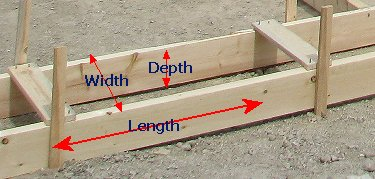Spike's Calculators

# Concrete Volume for Pouring FootingsCalculate the amount of concrete needed for pouring footings using feet and inches for measurements.

### Concrete Footings

Length of Footings ft in
Width in
Depth of Footings in

Perimeter of Footings ft

Cubic Feet ft³
Cubic Yards yd³
Cubic Metres

#### Calculation

1. enter the length of the footings in feet and inches
2. the width in inches
3. depth of footings in inches

#### Results

1. the perimeter of footings (for forming material in lineal feet)
2. amount of concrete needed in cubic feet
3. concrete needed in cubic yards
4. concrete needed in cubic metres
##### Formula
`length * width * depth = Volume`
##### Example calculation:
```length of footings  = 120'
width = 20"
depth of  = 6"
```

The multiplication is easier done if all the units used are the same.

Convert the inches to feet by dividing the inch amount by 12 (there are 12 inches in one foot);

```20 ÷ 12 =  1.67 (rounded to two decimals)
6 ÷ 12 =  0.5```

Now using the above formula, you can easily calculate the volume of the footings in cubic feet;

`120 * 1.67 * 0.5 = 100.2`
You need 100.2 cubic feet of concrete.
```To convert cubic feet to cubic yards multiply cubic feet * 0.037037037037037
To convert cubic feet to cubic metres multiply cubic feet * 0.028316846592
To convert cubic yards to cubic metres multiply cubic yards * 0.764554857984
```

### When Ordering Concrete

This concrete calculator will help you in estimating the amount of concrete needed for your project. The amount given as needed, does not include any waste. It is recommended, depending on the job you are doing, to add anywhere from an extra 4% to 10% to your concrete order to make sure you have enough concrete to finish the job. Ask when ordering the concrete. They will be able to give you a good idea of what is needed.